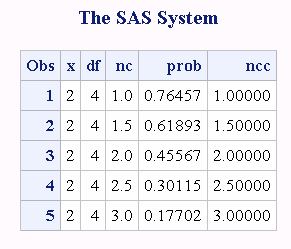# TNONCT Function

Returns the value of the noncentrality parameter from the Student's t distribution.

 Category: Mathematical

## Syntax

TNONCT(x,df,prob)

### Required Arguments

#### x

is a numeric random variable.

#### df

is a numeric degrees of freedom parameter.

 Range df > 0

#### prob

is a probability.

 Range 0 < prob < 1

## Details

The TNONCT function returns the nonnegative noncentrality parameter from a noncentral t distribution whose parameters are x, df, and nc. A Newton-type algorithm is used to find a root nc of the equation
$equation$
The following relationship applies to the preceding equation:
$equation$
If the algorithm fails to converge to a fixed point, a missing value is returned.

## Example

The following example computes the noncentrality parameter from the t distribution.
```data work;
x=2;
df=4;
do nc=1 to 3 by .5;
prob=probt(x,df,nc);
ncc=tnonct(x,df,prob);
output;
end;
run;
proc print;
run;```
Computations of the Noncentrality Parameter from the t Distribution